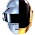## Contains Duplicate II

Given an array of integers and an integer k, find out whether there are two distinct indices i and j in the array such that nums[i] = nums[j] and the difference between i and jis at most k.

### Analysis:

Same idea as previous question that Hash table is used. The only difference if the requirement of minimum distance between the same elements is at most k.

Remember that previous question, we set the value of hash table True/False, in this question, we change that to the index of element. Therefore we can keep tracking the distance between the two elements. A simple check can solve the problem.

### Code(C++):

class Solution {
public:
bool containsNearbyDuplicate(vector<int>& nums, int k) {
if (nums.size() == 0 ){
return false;
}
map<int,int> mp;
for (int i=0;i<nums.size();i++){
if (mp.find(nums[i])==mp.end()){
mp[nums[i]] = i;
}else{
if (i - mp[nums[i]] <= k){
return true;
}else{
mp[nums[i]] = i; //Update the position
}
}
}
return false;
}
};


### Code(Python):

class Solution(object):
def containsNearbyDuplicate(self, nums, k):
"""
:type nums: List[int]
:type k: int
:rtype: bool
"""
if len(nums) == 0:
return False
mp = {}
for i in range(len(nums)):
# Note: here you cannot use "if not "
# because if mp[nums[i]] == 0, if not will also return True
if mp.get(nums[i]) == None:
mp[nums[i]] = i
elif (i - mp[nums[i]]) <= k:
return True
else:
mp[nums[i]] = i
return False


#### 1 comment:

1.public class Solution {
public boolean containsNearbyDuplicate(int[] nums, int k) {
HashMap map = new HashMap();
for (int i=0; i=k) {
return true;
}
map.put(nums[i], i);
}
return false;
}
}

The above was my java solution. It failed a test case with input ([99,99], 2). The expectation was true, but I think its wrong.